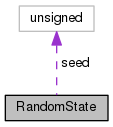RandomState Struct Reference

`#include <kaldi-math.h>`

Collaboration diagram for RandomState:[legend]

RandomState ()

unsigned seed

## Detailed Description

Definition at line 136 of file kaldi-math.h.

## ◆ RandomState()

 RandomState ( )

Definition at line 59 of file kaldi-math.cc.

References kaldi::Rand(), and RandomState::seed.

59  {
60  // we initialize it as Rand() + 27437 instead of just Rand(), because on some
61  // systems, e.g. at the very least Mac OSX Yosemite and later, it seems to be
62  // the case that rand_r when initialized with rand() will give you the exact
63  // same sequence of numbers that rand() will give if you keep calling rand()
64  // after that initial call. This can cause problems with repeated sequences.
65  // For example if you initialize two RandomState structs one after the other
66  // without calling rand() in between, they would give you the same sequence
67  // offset by one (if we didn't have the "+ 27437" in the code). 27437 is just
68  // a randomly chosen prime number.
69  seed = Rand() + 27437;
70 }
int Rand(struct RandomState *state)
Definition: kaldi-math.cc:45

## ◆ seed

 unsigned seed

Definition at line 138 of file kaldi-math.h.

Referenced by kaldi::Rand(), and RandomState::RandomState().

The documentation for this struct was generated from the following files: